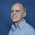## onsdag 16 oktober 2013

### The Higgs: Searching for an Elephant by Microscope

Modern physics is based on two supposedly incompatible theories for the four forces of physics acting on different scales
1. gravitational force: macroscopic: relativity theory: cosmology
2. electromagnetic, weak and strong forces: microscopic: quantum mechanics: atoms
The incompatibility has made a unified theory impossible and has driven modern physics into absurdities such as searching for the origin of macroscopic gravitation on microscopic atomistic scales.

The 2013 Nobel Prize for the Higgs particle falls into this tradition: The idea is that mass or matter as the subject of macroscopic gravitation is generated from subatomic interactions through the Higgs particle with the Higgs field as an endless ocean in which the Universe is floating.

Since the manifestation of mass is gravitation, it means to search for macroscopics in microscopics, that is searching for an Elephant using a microscope (quantum loop gravity and string theory) . It does not seem to me to be a constructive approach.

A different approach is sketched in my pet theory described in Newtonian Gravitation of Matter and Antimatter exploring the possibility that
• the basic element of the Universe is a gravitational field $\phi (x,t)$ depending on a space coordinate $x$ and time coordinate $t$
• matter and antimatter of density $\vert\rho (x,t)\vert$ is created by differentiation with respect to $x$ of the field $\phi (x,t)$ through the Laplace operator $\Delta$: $\rho =\Delta\phi$ with matter where $\Delta\phi >0$, antimatter where $\Delta\phi < 0$ and vaccum where $\Delta\phi =0$
• a gravitational force $F$ arises as the space gradient $\nabla$ of the field $\phi$: $F = \nabla\phi$.
It is thus the gravitational field $\phi$ which
• creates visible matter where $\Delta\phi$ is positive and singular
• creates visible antimatter where $\Delta\phi$ is negative and singular
• creates dark matter and antimatter where $\Delta\phi$ is smooth
• separates matter and antimatter by gravitational repulsion
• concentrates matter by gravitational attraction.
What about that? Hint: The Hen and the Egg of (Dark) Matter. This is something completely different from the Higgs as an "explanation" of the origin of mass.

#### 1 kommentar:

1.More simply: The Higgs particle has an energy in the 100 GeV range, with a mass equivalence three orders of magnitude times the mass of the hydrogen atom. Imagine two hydrogen atoms, supposedly gravitationally bound by passing "something" 1,000 times their own mass--or energy--back and forth between them. Complete lunacy.